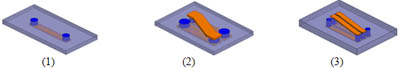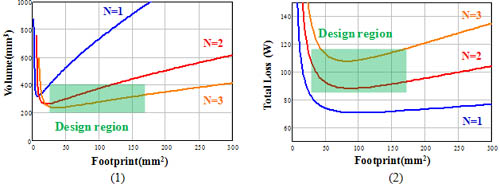Contact Us
LIBRARY

# Design Optimization of Non-uniform Flux LTCC Inductor Substrate for 3D Integrated POLFig. 1. LTCC lateral flux inductor. (1) 1-turn structure (N=1); (2) 2-turn structure (N=2); (3) 3-turn structure (N=3).
In order to reduce the converter footprint and fully use space, 3D integration concepts are widely used in POL converters, which design the magnetic component as a low-profile substrate. Low Temperature Co-fired Ceramics (LTCC) technology a prospective candidate for low-profile magnetic integration. LTCC is a relatively mature technology for RF applications due to its favorable thermo-mechanical properties when integrated with silicon. Also, it is easy to build thick conductor with LTCC technology for high current applications. Fig.1 shows some examples of LTCC lateral flux inductor with different structures. In these LTCC inductors, silver paste is screen printed into via to build via conductor; Copper is used to build surface coil. Because the flux distribution is very non-uniform for these lateral flux LTCC inductor structure, the conventional inductance and core loss calculation method for discrete inductor, which assumes flux is uniform, cannot be used directly.

This LTCC magnetic material also has very non-linear permeability, which makes this problem even more unconventional and complicate. A inductor model was proposed by CPES to calculate inductance and core loss for these LTCCC inductor by dividing disc core into many concentric rings. Based on proposed inductor model, inductor footprint, volume and loss can be predicted and considered together to optimize the low profile LTCC inductor design. Fig.2 shows design curves for LTCC planar inductor. Fig.2(a) shows the impact of inductor footprint on inductor volume with give inductance value (L=300nH). it can be seen that 1) increasing turn number can reduce inductance volume. 2) there is an optimal footprint to give minimal inductor volume. Fig.2(b) shows the impact of inductor footprint on inductor loss with give inductance value (L=300nH). It can be seen that 1) increasing turn number will increase inductor total loss 2) when footprint is small, increasing footprint will reduce inductor loss, but after some point, further increasing footprint will not impact inductor loss too much. According to Fig.2, it can be seen that there is a footprint region to achieve small inductor volume and loss at the same time. For this case, the preferred footprint region should be from 50mm2 to 100mm2.Fig. 2. Design curves for LTCC lateral flux inductor.
INDUSTRY PARTNERS